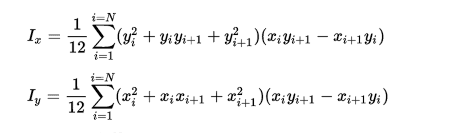# Moment of Inertia for Simple Polygon

• Jack2927

#### Jack2927

I am trying to determine if a function I have is working correctly. The function computes the moment of inertia for a polygon. To test the function I created a polygon that has 4 points and represents a square of 4 units by 4 units. The answer I am getting is 32. I believe the answer should be 42.6 based on J0= Ixx +Iyy, where Ixx = (bh^3)/12 and Iyy = (hb^3)/12.

The function I have is implementing the following.Which is correct 32 or 42.6?